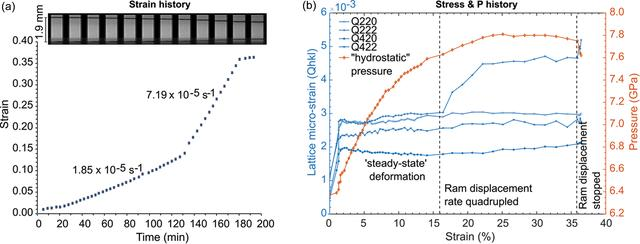disable zoom     view article Figure 11 Deformation of NaCl at high pressure and room temperature. (a) Strain history was obtained by X-ray radiography. The black lines in the snapshot images are Pt metal foil disks between the sample and the alumina pistons and indicate the shortening (i.e. strain) in the sample at constant displacement rate of the hydraulic rams (1 and 2). The strain increments (blue points) were calculated as a function of time from each radiography image. The error in the strain estimation of each step is approximately ±0.0013 (symbol size). The data are fitted by linear functions to obtain the strain rate at each deformation step. (b) Diffraction data of NaCl (data points), obtained after each capture of a radiographic image are evaluated for Q(hkl), a representation of the lattice micro-strain (i.e. stress) for selected hkl, and plotted against strain. The pressure history during deformation is calculated from dP(hkl) using the equation of state in the work by Brown (1999). While random errors in Q(hkl) and dP(hkl) are expected to be low in the data, large systematic errors are expected (using only two Ge-SSDs) and are not evaluated here.JOURNAL OFSYNCHROTRONRADIATION
ISSN: 1600-5775
Volume 29| Part 2| March 2022| Pages 409-423